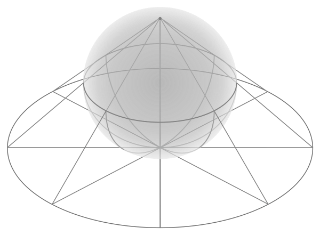# Geometry

Topic | v1 | created by janarez |Description

Geometry (from the Ancient Greek: γεωμετρία; geo- "earth", -metron "measurement") is, with arithmetic, one of the oldest branches of mathematics. It is concerned with properties of space that are related with distance, shape, size, and relative position of figures. A mathematician who works in the field of geometry is called a geometer. Until the 19th century, geometry was almost exclusively devoted to Euclidean geometry, which includes the notions of point, line, plane, distance, angle, surface, and curve, as fundamental concepts.During the 19th century several discoveries enlarged dramatically the scope of geometry. One of the oldest such discoveries is Gauss' Theorema Egregium (remarkable theorem) that asserts roughly that the Gaussian curvature of a surface is independent from any specific embedding in an Euclidean space.

##### Relations

Euclidean geometry is a mathematical system attributed to Alexandrian Greek mathematician Euclid, whi...